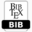AUTHOR(S): Doretta Vivona, Pierre Capodanno TITLE Mathematical analysis of the small oscillations of a bubble in a cylindrical liquid column under gravity zeroPDFBIBTEX ABSTRACT This paper deals with the mathematical study of the small oscillations of a system formed by a a cylindrical liquid column bounded by two parallel circular disks and an internal spherical bubble constitued by a barotropic gas, under zero gravity. From the equations of motion, the authors deduce a variational equation. Then the study of the small oscillations depends on the coerciveness of a hermitian form that appears in this equation. It is proved that this last problem is reduced to an auxiliary eigenvalue problem. A careful discussion shows that our problem is a classical vibration problem. KEYWORDS Free Boundaries, small oscillations, variational methods. REFERENCES  H.F Bauer, Natural frequencies and stability of circular cylinderical immiscible, Int.J.Microgravity. Res. Appl. 2, 1989, pp. 27- 44.  H.F Bauer, Natural frequencies and stability of circular cylinderical immiscible, Int.J.Microgravity. Res. Appl. 2, 1989, pp. 27- 44.  H.F. Bauer and W. Eidel, Linear liquid oscillations in a cylindrical container under zero gravity, Microgravity Sci. and Techn. 2, 1990, pp.212-220.  P. Capodanno, Small oscillations of a liquid in a container of revolution under zero gravity, Eur.Jour.Mech. B-Fluids- 11-3, 1992, pp. 291- 308.  P. Capodanno, On the small oscillations of a catenoidal liquid bridge between two parallel ´ plates under zero gravity, Microgravity Science and Tecnology 7-3, 1994, pp. 252-257.  P. Capodanno, Sur les petites oscillations d’une gutte de liquid catenoidale entre deux plaques paralleles au apesanteur, Zeitschrift fur ange- ¨ wandte Mathematik und Physik (Z.A.M.P.), 48, 1995, pp. 724-738.  P. Capodanno, Mathematical study of the small oscillations of a liquid column in a symmetrical cylindrical container under zero gravity, Jour. Fluids and Struct., 9, 1995,pp. 773-786.  P. Capodanno and D. Vivona, Small oscillations of an anviscid liqud in a rigid container under zero gravity, when the conctat line is fixed, Ricerche di Matematiche, 50-1, 2001, pp. 35-51.  P. Capodanno and D. Vivona, Mathematical study of the small oscillations of an incompressible and inviscid liquid under zero gravity in a container of revolution with elastic bottom and anchored edges, Ann.Un.Ferrara, 48-7, 2002, pp. 25-47.  P. Capodanno and D. Vivona, On a variational equation of the small oscillations of a bubble in a cylindrical liquid column under gravity zero, Proc. 12th Int.Congress on Fluid Mechanics and Aerodynamics (FMA’14) Geneve, 2014.  R. Dautray and J.–L. Lion, Analyse mathematique et Calcul num ´ erique ´ , 5, Masson–Paris, 1988.  N.–D. Kopachevsky and S.–G. Krein,Operator aproach to linear problems of hydrodynamics, 1, Birka¨user–Basel, 2001.  H. Lamb, Hydrodynamics, Cambridge University Press, Cambridge, 1932.  L. Landau and E. Lifschitz, Mecanique des Flu- ´ ides, Editions MIR, Moscou, 1971.  D. Langbein, Stability of liquid bridge between parallel plates, Microgr. Sci.and Techn., 5, 1995,pp.2-11.  D. Langbein, F. Falk and R. Grossbach, Oscillations of fluid column under microgarvity.Adv.Space.Res., 16-7, 1995, pp.23-26.  N.–N. Moiseyev and V.–V. Rumjantsev, Dynamic stability of bodies containing fluid, Springer –Verlag, Berlin, 1968.  M.–J.-P. Morand and R. Ohayon, Interactions fluides-structures, Masson, Paris, 1992.  M. Roseau, Vibration des systemes mecanique ´ , Masson Paris, 1984.  Hubert J. Sanchez and Palencia E. Sanchez, Vibration and coupling of continuous systems; asymptotic methods, Springer Berlin, 1989.  L. Schwartz, Theorie des didtributions ´ , Hermann, Paris, 1966.  D. Vivona, Mathematical study of the small oscillations of a spherical liquid bridge between two equal disks under gravity zero, Bull.Polish Acc. Sc., 49-1, pp.31-49, 2001.  T.I. Vogel, Stability of a liquid drop trapped betwen two parallel plates. S.I.A.M. J.Appl.Mech., 47-3, 1987, pp.516-525. Cite this paper Doretta Vivona, Pierre Capodanno. (2016) Mathematical analysis of the small oscillations of a bubble in a cylindrical liquid column under gravity zero. International Journal of Mathematical and Computational Methods, 1, 92-98Copyright © 2016 Author(s) retain the copyright of this article.This article is published under the terms of the Creative Commons Attribution License 4.0